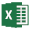### 7.3.2. Bending Stiffness

Reference: Abbott, Richard. Analysis and Design of Composite and Metallic Flight Vehicle Structures 3 Edition, 2019

7.3.2.1. Simple Cantilever

The expression will be given in terms of stiffness per unit width:

Where:

The expression for stiffness per unit width of beam can easily be derived as

7.3.2.2. Guided Cantilever

The expression will be given in terms of stiffness per unit width:

The expression for Stiffness can easily be derived asAA-SM-260 Tools – Spring Stiffness of Cantilever Beams

### 7.3.2. Bending Stiffness

Reference: Abbott, Richard. Analysis and Design of Composite and Metallic Flight Vehicle Structures 3 Edition, 2019

7.3.2.1. Simple Cantilever

The expression will be given in terms of stiffness per unit width:

Where:

The expression for stiffness per unit width of beam can easily be derived as

7.3.2.2. Guided Cantilever

The expression will be given in terms of stiffness per unit width:

The expression for Stiffness can easily be derived asAA-SM-260 Tools – Spring Stiffness of Cantilever Beams